Adding Calculations to a SeamlessDocTo run calculations on a SeamlessDoc:

Select the text field that you'd like to show the total your calculation. Then, click the Calculations button on the field toolbar which will look like a calculator: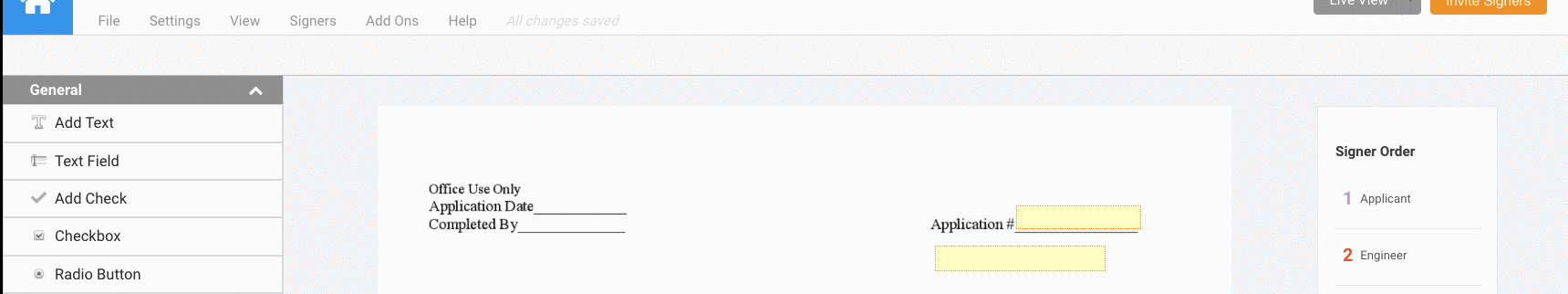The field toolbar will be replaced with a function bar into which you can write out your mathematical expression. Here is an example of what an expression could look like: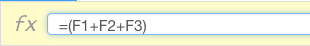• Please keep in mind that you must keep the ‘=’ (equals) symbol at the beginning of any of the equations. You also want to make sure to delete any spaces in the expression.

All of the applicable fields will appear with a unique cell label like F1, F2, F3 etc. Each of these F#’s can be thought of as an individual cell in an application such as Excel.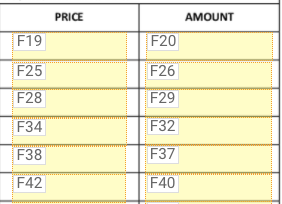Using the cell label you can create formulas to calculate the data entered into these fields to be populated into the field where you clicked on the calculations button. For example in this image below, I want to create a formula where all of my values under Tax Due are totaled into the Amount of Tax Due field.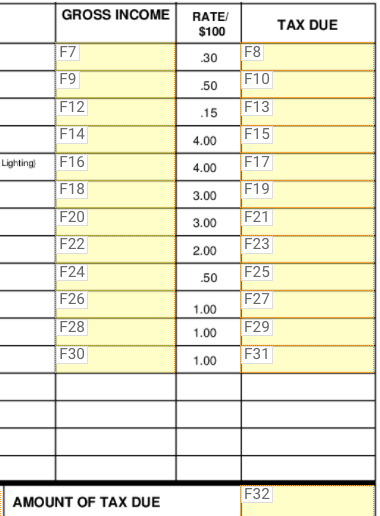The proper formula would look like this: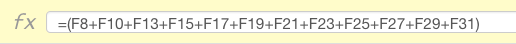Here is more advanced information about the different functions that can be used in a formula for calculations:

Algebraic Functions

SeamlessDocs recognizes order of operations, so keep in mind that certain algebraic functions will execute before others. A great way to remember to order of operation is the acronym PEMDAS:

Parentheses → Exponents → Multiplication → Division → Addition → Subtraction

Regardless of the arrangement of the algebraic expression from left to right, functionality related to parentheses is always going to coming before exponents, which is going to come before multiplication, and so on. Check out an example below:

“DOLLAR” Formatting

In a Web Form, you can add the function “DOLLAR” so that the end value calculated into a given field will appear in standard currency formatting (see image below). All fields that make use of the “DOLLAR” before your calculation will appear with a USD dollar sign, applicable commas, and two figures for cents. Conversely, if you are in a form that you have converted from a PDF (SeamlessDoc) you can also format any text field for dollar formatting by going into the More Options button on a field.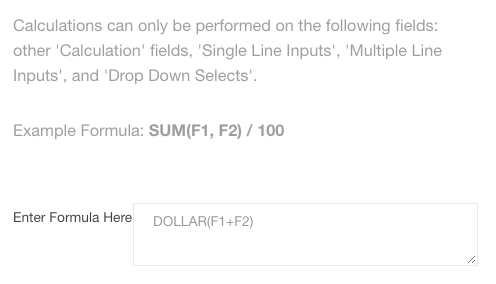“ROUND” Function

By adding the function “ROUND” directly after the ‘=’ (equals) symbol, the end value will round to whatever number of decimal places you set. The format will look like this f=ROUND(Cell Label, # of Decimal places set):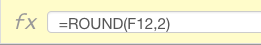* Make sure to put an open parenthesis after the word “ROUND”.

IF/THEN Statements

All IF/THEN Statements should be formatted as follows:

IF (Condition, Value if True, Value if False)

• A Condition is any True or False Statement. For example, you can compare a field to a value such as F1 > 5 or a field to another field such as F1 = = F2
• The Value if True means if the condition is true, the field will display that first value.
• The Value if False means that if the condition is false, it will display the second value.
• In the example above if someone were type in a number greater than 100 then 500 will appear in the field with the calculation, if they put in a number lass than 100 then the number 300 will appear in the field with calculations.

Adding Static Values to Checkboxes, Radio Buttons and Select Menus

- Checkboxes and Radio Buttons -

To add a static values to Checkboxes or Radio Buttons, go into the Advanced Field Settings for either of the field types by clicking on the gear icon on the right of the field toolbar: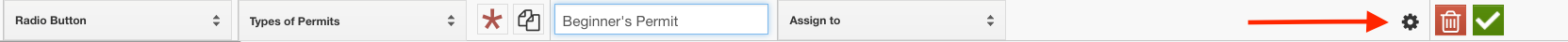From the Advanced Field Settings, you’ll be able to enter a static value into the “Calculation Field” section. If and when any Checkboxes or Radio Buttons are clicked, the corresponding static values will apply within any calculations into which the fields were added.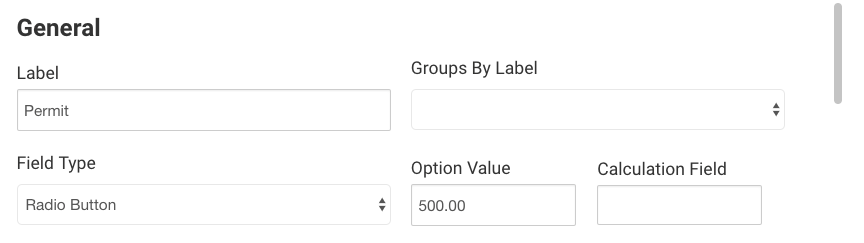- Select Menus -

To add static values to Select Menus, go to the Advanced Field Settings by clicking on the gear icon on the right of the field toolbar: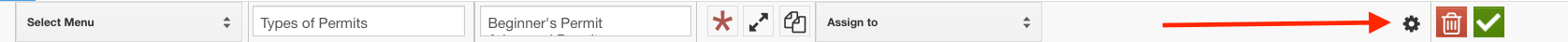From the Advanced Field Settings, you’ll be able to enter a static values that associate with each of the options within the Select Menu. The numbers entered in the right-hand box correlate horizontally with the name of the option in the left-hand box.